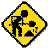# Dining Philosophers

This example is still under construction.This site contains some screenshots of the following example. The presented GUI was generated automatically.
```type Philosopher = Int
data Mode = Think | Eat
data Fork = Up (Philosopher) | Down

instance AsmTerm (Philosopher -> Rule ())

instance AsmTerm Mode where
asmDefault = Think

instance AsmTerm Fork where
asmDefault = Down

mode :: Dynamic (Philosopher -> Mode)
mode = initAssocs "mode" []

fork :: Dynamic (Philosopher -> Fork)
fork = initAssocs "fork" []

acts :: Dynamic (Philosopher -> (Philosopher -> Rule ()))
acts = initAssocs "acts" []

output :: Dynamic (Philosopher -> String)
output = initAssocsStdout False "output"

exec :: Philosopher -> Rule ()
exec self =
if mode(self)==Think && lfork==Down && rfork==Down then do
lfork := Up(self)
rfork := Up(self)
mode(self)   := Eat
output(self) := "Philosopher " ++ show self ++ " is eating\n"
else if mode(self)==Eat then do
lfork      := Down
rfork      := Down
mode(self) := Think
else skip
where lfork  = fork(self)
rfork  = fork(right)
right  = (self+1) `mod` card(dom(acts))
left   = (self-1) `mod` card(dom(acts))

initAgents :: Rule ()
initAgents = forall p <- [0..4] do
acts(p) := exec

main :: Rule ()
main = multi acts
```